SLUS515G September   2002  – December 2015

PRODUCTION DATA.

1. Features
2. Applications
3. Description
4. Revision History
5. Pin Configuration and Functions
6. Specifications
7. Detailed Description
1. 7.1 Overview
2. 7.2 Functional Block Diagram
3. 7.3 Feature Description
4. 7.4 Device Functional Modes
8. Application and Implementation
1. 8.1 Application Information
2. 8.2 Typical Application
1. 8.2.1 Design Requirements
2. 8.2.2 Detailed Design Procedure
3. 8.2.3 Application Curves
9. Power Supply Recommendations
10. 10Layout
1. 10.1 Layout Guidelines
2. 10.2 Layout Example
11. 11Device and Documentation Support
12. 12Mechanical, Packaging, and Orderable Information

#### Package Options

Refer to the PDF data sheet for device specific package drawings

• D|8
• P|8

## 8 Application and Implementation

NOTE

Information in the following applications sections is not part of the TI component specification, and TI does not warrant its accuracy or completeness. TI’s customers are responsible for determining suitability of components for their purposes. Customers should validate and test their design implementation to confirm system functionality.

### 8.1 Application Information

The UCC38050 and UCC38051 are switch-mode controllers used in boost converters for power factor correction operating in transition mode. In the transition mode operation, the PWM circuit is self-oscillating, with the turnon being governed by an inductor zero-current detector (ZCD pin), and the turnoff being governed by the current-sense comparator. Additionally, the controller provides features such as peak current limit, default timer, OVP, and enable.

There are two key parametric differences between UCC38050 and UCC38051. The UVLO turnon threshold of UCC38050 is 15.8 V, while for UCC38051 it is 12.5 V. Secondly, the gM amplifier source current for UCC38050 is typically 1.3 mA, while for UCC38051 it is 300 μA. The UCC38051 is suitable for multiple applications, including AC adapters, where a two-stage power conversion is needed. The UCC38050 is suitable for applications such as electronic ballasts, where there is no down-stream PWM conversion and the advantages of a smaller VCC capacitor and improved transient response can be realized. Figure 19 is an example of a critical conduction mode power factor correction boost converter utilizing the UCC38050.

### 8.2 Typical Application

The UCC38050 is used for the off-line power factor corrected pre-regulator with operation over a universal input range of 85 V to 265 V with a 400-VDC regulated output. The schematic is shown in Figure 19, and the board layout for the reference design is shown in Figure 24.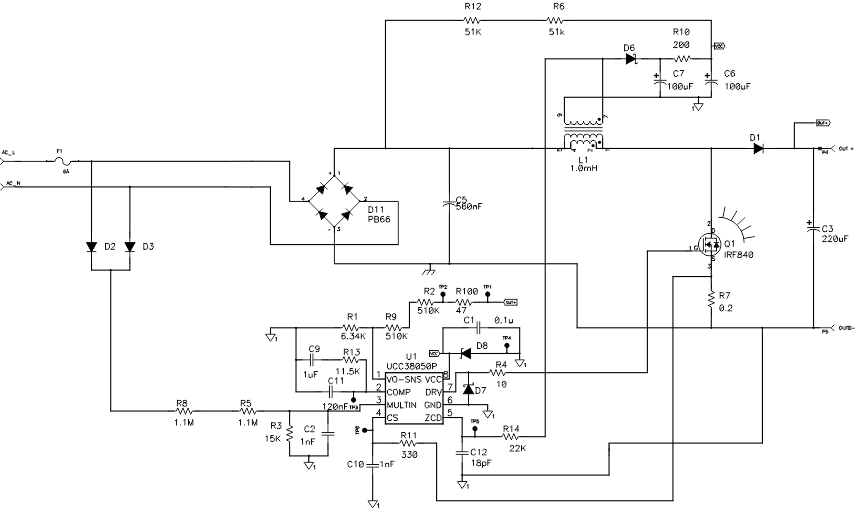Figure 19. Universal Line Input 100-W Boost Converter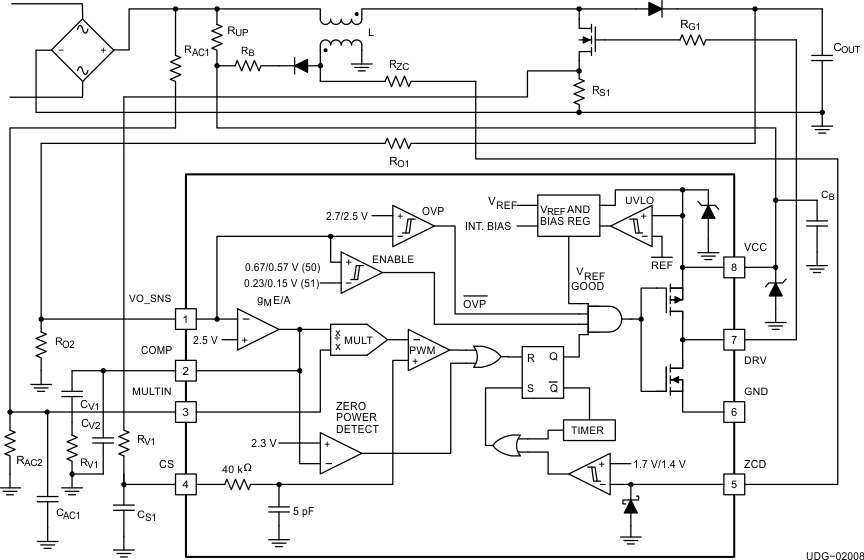Figure 20. Typical Application Diagram

#### 8.2.1 Design Requirements

Table 1 shows the design requirements for a CCM, PFC boost converter utilizing the UCC38050.

### Table 1. UCC38050 Design Requirements

PARAMETER TEST CONDITION MIN TYP MAX UNIT
VIN Input voltage 85 265 VRMS
Input frequency 60 Hz
VOUT Output voltage DC VIN = 85 VRMS 370 400 425 V
VOUT Output voltage DC VIN = 265 VRMS 370 390 410 V
POUT Output power 0 100 W
Output voltage ripple VIN = 85 VRMS 3%
Efficiency POUT = 100 W 90%
Total harmonic distortion (THD) VIN = 85 VRMS, POUT = 100 W 5%
Total harmonic distortion (THD) VIN = 265 VRMS, POUT = 100 W 15%
Hold-up time 16.7 ms

#### 8.2.2 Detailed Design Procedure

For a selected VOUT and minimum switching frequency, the following equations outline the design guidelines for power stage component selection, using a universal input, 100-W PFC converter with an output voltage of 390 V. Refer to Figure 20 for reference designators.

#### 8.2.2.1 Inductor Selection

In the transition mode control, the inductor value must be calculated to start the next switching cycle at zero current. The time it takes to reach zero depends on line voltage and inductance and as shown in Equation 2. L determines the frequency range of the converter.

Equation 2.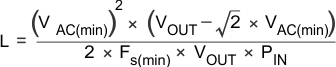where

• VAC = RMS line voltage
• VAC(min) = minimum AC line voltage
• PIN = maximum input power averaged over the ac line period
Equation 3. IL(peak) = 2 × √2 × (PIN/VAC(min))
Equation 4. IL(rms) = IL(peak) / √6

#### 8.2.2.2 MOSFET Selection

The main switch selection is driven by the amount of power dissipation allowable. Choose a device that minimizes gate charge and capacitance, and minimizes the sum of switching and conduction losses at a given frequency.

Equation 5.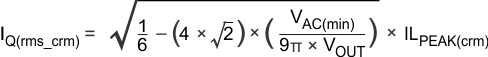Equation 6. VQ(max) = VOUT

#### 8.2.2.3 Diode Selection

The effects of the reverse recovery current in the diode can be eliminated with relatively little negative impact to the system. The diode selection is based on reverse voltage, forward current, and switching speed.

Equation 7. ID(avg) = IOUT(avg)
Equation 8.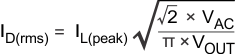Equation 9. VD(peak) = VOUT

#### 8.2.2.4 Capacitor Selection

The hold-up time is the main requirement in determining the output capacitance. ESR and the maximum RMS ripple current rating can also be important, especially at higher power levels.

Equation 10. COUT(min) = (2 × POUT × tHOLDUP) / ((VOUT)2 – (VOUT(min))2)

where

• VOUT(min) = minimum regulator input voltage for operation
Equation 11.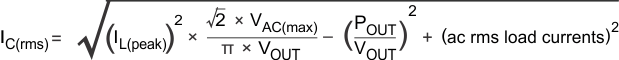#### 8.2.2.5 Multiplier Set-Up

Select RAC1 and RAC2 so that their ratio uses the full dynamic range of the multiplier input at the peak line voltage, and yet with values small enough to negate the effects of the multiplier bias current. To use the maximum range of the multiplier, select the divider ratio so that VMULTIN, evaluated at the peak of the maximum ac line voltage, is the maximum of the minimum dynamic input range of MULTIN, which is 2.5 V. Choose RAC1 so that it has at least 100 μA at the peak of the minimum AC operating line voltage.

Equation 12.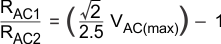In extreme cases, switching transients can contaminate the MULTIN signal, so it can be beneficial to add capacitor CAC1. Select the value of CAC1 so that the corner frequency of the resulting filter is greater than the lowest switching frequency. The low corner frequency of this filter may compromise the overall power factor.

#### 8.2.2.6 Sense Resistor Selection

The current sense resistor value must be chosen to limit the output power, and it must also use the full dynamic range of the multiplier during normal steady state operation. The value of RS1 is thus selected for maximum power operation at low ac line voltage conditions. To use the full dynamic range, set the VSENSE threshold as a function of the dynamic input range of VCOMP and the peak of the minimum MULTIN voltage.

Equation 13.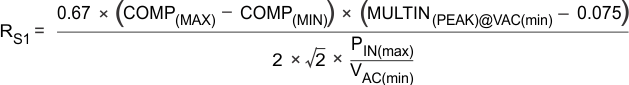where

• COMP(MAX) = 3.8 V
• COMP(MIN) = 2.5 V
• MULTIN(PEAK)@VAC(min) = √2 × VAC(min)( RAC2 / (RAC2+RAC1) )

If the exact value RS1 is not available, RS2 and RS3 can be added for further scaling. The CS pin already has an internal filter for noise due to switching transients. Additional filtering at switching transient frequencies can be achieved by adding CS1.

#### 8.2.2.7 Output Voltage Sense Design

Select the divider ratio of RO1 and RO2 to set the VO_SNS voltage to 2.5 V at the desired output voltage. The current through the divider should be at least 200 μA.

#### 8.2.2.8 Voltage Loop Design

How well the voltage control loop is designed directly impacts line current distortion. UCC38050 employs a transconductance amplifier (gM amp) with gain scheduling for improved transient response (refer to Figure 9). Integral type control at low frequencies is preferred, because the loop gain varies considerably with line conditions. The largest gain occurs at maximum line voltage. If the power factor corrector load is dc-to-dc switching converter, the small signal model of the controller and the power factor corrector, from COMP to PFC output voltage is given by:

Equation 14.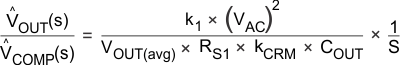where

• ^VOUT = small signal variations in VOUT
• ^VCOMP = small signal variations in VCOMP
• k1 = multiplier gain = 0.65
• kCRM = peak to average factor = 2

A controller that has integral control at low frequencies requires a zero near the crossover frequency to be stable. The resulting gM amplifier configuration is shown in Figure 21.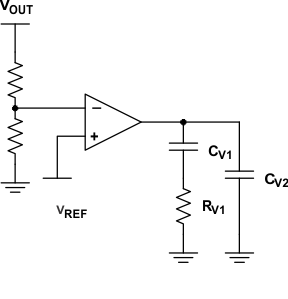Figure 21. gM Amplifier Configuration

The compensator transfer function is:

Equation 15.where

• gM = DC transconductance gain = 100 μs

The limiting factor of the gain is usually the allowable third harmonic distortion, although other harmonics can dominate. The crossover frequency of the control loop will be much lower than twice the AC line voltage. To choose the compensator dynamics, determine the maximum allowable loop gain at twice the line frequency, and solve for capacitor CV2. This also determines the crossover frequency.

Equation 16.Equation 17.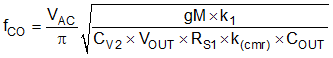Select CV1 so that the low frequency zero is one-tenth of the crossover frequency.

Equation 18. CV1 = 9 CV2

Select RV1 so that the pole is at the crossover frequency.

Equation 19. ≈ 1 / 2π fCO CV2

#### 8.2.3 Application Curves

Figure 22 and Figure 23 show the input current and rectified line for the power module.

• Channel 3 = Rectified Line Voltage
• Channel 4 = Power Module Input CurrentVIN = 85 V POUT = 100 W
Figure 22. Rectified Line Voltage and Power Module Input Current at 85 V/100 W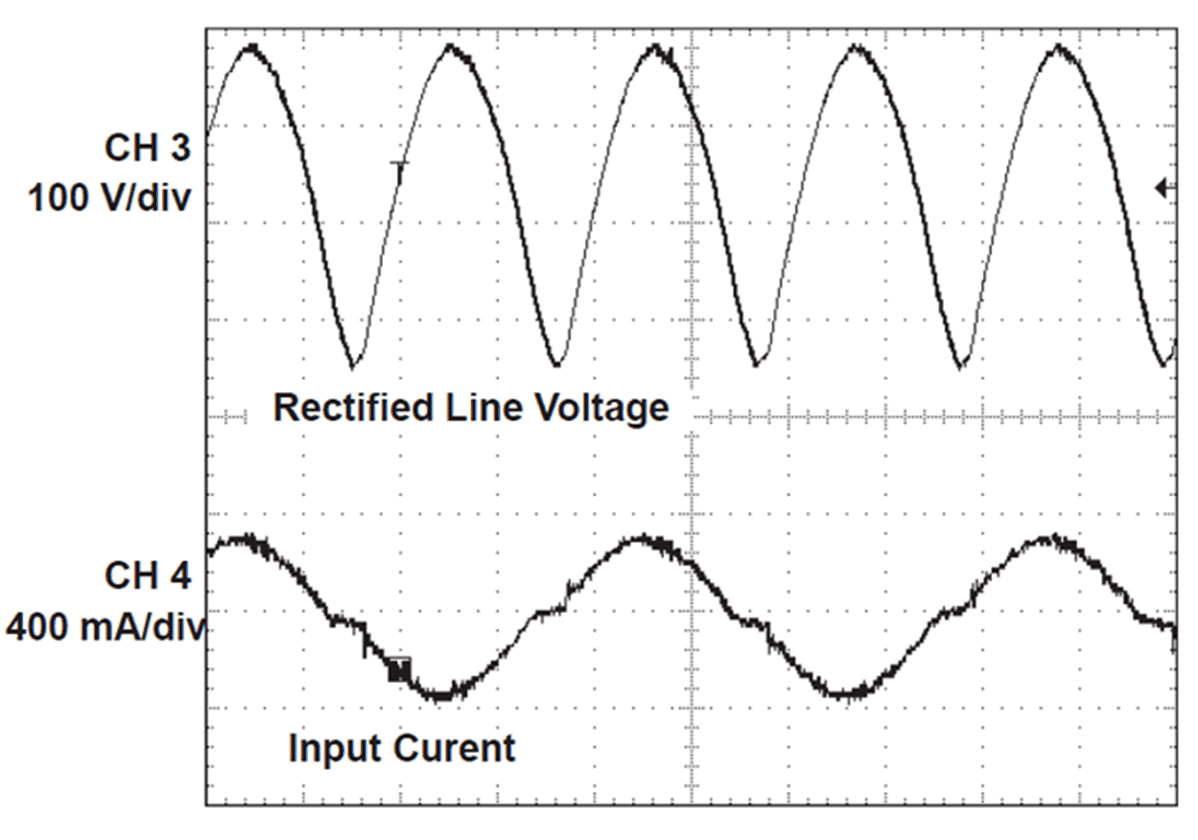VIN = 265 V POUT = 100 W
Figure 23. Rectified Line Voltage and Power Module Input Current at 265 V/100 W